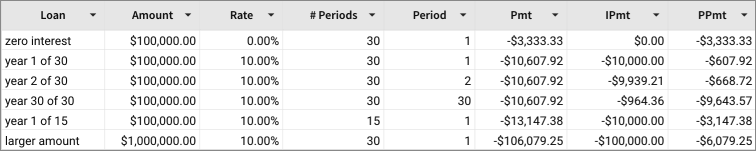# PPmt

The PPmt function returns the principal portion of a periodic, constant payment for an investment or loan with a constant interest rate.

To determine the total payment, or how much is allocated to interest, use the Pmt and IPmt functions.

PPmt is part of the set of financial functions that Sigma supports.

## Syntax

`PPmt(rate, period, nperiods, pv, [fv], [type])`

The PPmt function has the following arguments:

rate
Required.
The interest rate for the loan.
period
Required.
Current payment period.
The valid range is 1 through `nperiods`.
nperiods
Required.
The total number of payments for the loan.
pv
Required.
The present value, or total value of all loan payments; the amount borrowed.
fv
Optional.
The future value, or a cash balance you want after the last payment is made.
Defaults to 0 (zero).
type
Optional.
When payments are due:
0
End of period
1
Beginning of period
Default is 0.

## Notes

• Be consistent with the units for rate and nperiods arguments. If you make monthly payments on a two-year loan at an annual interest rate of 7%, use the rate calculation of 0.07/12 and nperiods calculation of 2*12. For annual payments on the same loan, use the rate of 0.07 and nperiods of 2.

## Examples

`PPmt(.07/12,1,2*12,10000)PPmt(.07/12,2*12,2*12,10000)`

The first monthly interest payment for a loan of \$10,000, with an annual interest rate of 7% is \$389.39. The last (24th) interest payment is \$445.13.

`PPmt(.07,1,2,10000)PPmt(.07,2,2,10000)`

The first year's interest payment for a two-year loan of \$10,000, with an annual interest rate of 7% is \$4,830.92. The last payment (second year) has the interest payment of \$5,169.08.The first yearly payment for a loan of \$100,000, with an annual interest rate of 10% over 30 years, compounded yearly, has the principal payment of \$607.92. The last payment (year 30) has the principal payment of \$9,643.57.